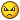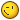# create an oop grid array ?

## Recommended Posts

hi,

i know create an oop object with a simple graphic shape like this :

`````` // create an new instance of a pixi container
var container = new PIXI.Container();
// create a renderer instance
var renderer = PIXI.autoDetectRenderer(320, 480);

// add the renderer view element to the DOM
document.body.appendChild(renderer.view);

requestAnimationFrame( animate );

var T={}
T.draw = function() {
PIXI.Graphics.call(this)
this.beginFill(0xFFFFFF)
this.drawRect(100,100,80,200)
}

T.draw.prototype = Object.create(PIXI.Graphics.prototype);
T.draw.prototype.constructor = T.draw;

var button=new T.draw()

function animate() {

requestAnimationFrame( animate );
renderer.render(container);
}``````

but I am lost on the fact of doing the same with  an 2d array. Can you point me ?

``````var graphics = [];
for (var j = 0; j < 5; j++) {
graphics[j] = [];
for (var i = 0; i < 5; i++) {
graphics[j][i] = new PIXI.Graphics();

graphics[j][i].beginFill(0xFF3300);
graphics[j][i].lineStyle(4, 0xffd900, 1);
graphics[j][i].drawRect(0,0,10,10);
graphics[j][i].position.x = 40 * i;
graphics[j][i].position.y = 40 * j;
};
};``````

##### Share on other sites

Edited sorry. Have you an idea to make this grid like an oop object. I have no idea to implement this due the 2 values.

##### Share on other sites

``````function Grid() {
PIXI.Container.call(this);
var graphics = [];
for (var j = 0; j < 5; j++) {
graphics[j] = [];
for (var i = 0; i < 5; i++) {
graphics[j][i] = new PIXI.Graphics();

graphics[j][i].beginFill(0xFF3300);
graphics[j][i].lineStyle(4, 0xffd900, 1);
graphics[j][i].drawRect(0,0,10,10);
graphics[j][i].position.x = 40 * i;
graphics[j][i].position.y = 40 * j;
};
};
this.graphics = graphics;
}

Grid.prototype = Object.create(PIXI.Container.prototype);
Grid.prototype.constructor = Grid;``````

Though i dont recomment use graphics, better to create texture and then sprites from them. "var tex = renderer.generateTexture(graphics)" will help you with that##### Share on other sites

hi @ivan.popelyshev, thanks for your snippet but i have this error :

``TypeError: child is undefined ``

at line :

``this.addChild(graphics[j][i]);``

for the texture, i use always but for the forum is use graphics, it is easier to test for others.

##### Share on other sites

@ivan.popelyshev just out of interest, is there any perf gain from using a local variable and then assigning that to the object after you've finished iteration? I've never seen it before, or did it just end up like that from quickly replying?

##### Share on other sites

ok i have seen my error i forgot to call the prototype by

var array=new Grid() in place of var array=Grid()

Is it possible to make the same ( a little like mattstyles say) like this :

(but as a good newbie this don't work)...

could you be say where is my error ?....

``````function Grid() {
var rectangle=PIXI.Graphics()
rectangle.beginFill(0xFF3300)
rectangle.drawRect(0,0,40,40)

PIXI.Graphics.call(this)
var grid=[];
for (var j = 0; j < 5; j++) {
grid[j] = [];
for (var i = 0; i < 5; i++) {
grid[j][i] = rectangle;
};
};
}

Grid.draw.prototype = Object.create(PIXI.Graphics.prototype);
Grid.draw.prototype.constructor = Grid;

var array=new Grid()``````

##### Share on other sites

Its only one rectangle that you are adding over and over again. I dont know what are you expecting

##### Share on other sites

i want to describe a variable (rectangle).

next i want use this rectangle in each cell of my grid.

##### Share on other sites

and why is it same rectangle? how do you think it will know that position differs for rectangles?

##### Share on other sites

thanks for the tips.

i have found the solution :

have a nice day``````function drawre() {
var rectangle=new PIXI.Graphics()
rectangle.beginFill(0xFF3300)
rectangle.drawRect(0,0,40,40)
return rectangle
}

function Grid() {
PIXI.Container.call(this)
var grid=[];
for (var j = 0; j < 5; j++) {
grid[j] = [];
for (var i = 0; i < 5; i++) {
grid[j][i] = drawre();
grid[j][i].x = i*50
grid[j][i].y =j*50

};
};

this.grid = grid

}

Grid.prototype = Object.create(PIXI.Container.prototype);
Grid.prototype.constructor = Grid;``````

##### Share on other sites

Yep, now its correct.

Are you coding in notepad? Either download sublime text editor and learn how to use it properly, either buy https://www.jetbrains.com/webstorm/ . My eyes hurt to see your formatting.

##### Share on other sites

i understand, normally i'm on vim but i'm at desk and i have no vim on my hand.

## Join the conversation

You can post now and register later. If you have an account, sign in now to post with your account.
Note: Your post will require moderator approval before it will be visible.×   Pasted as rich text.   Paste as plain text instead

Only 75 emoji are allowed.

×   Your previous content has been restored.   Clear editor

×   You cannot paste images directly. Upload or insert images from URL.